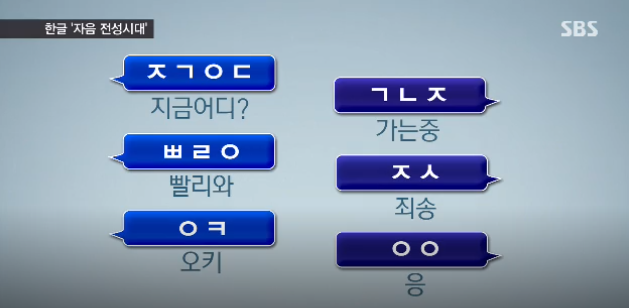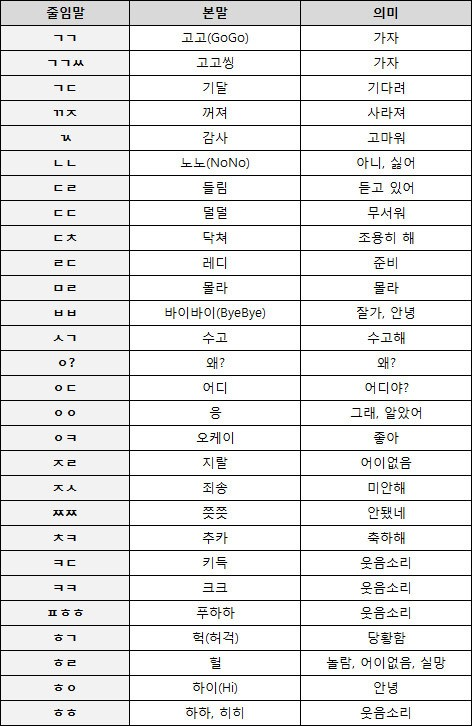(ㅈㄱㅇㄷ) ，ㄱㄴㅈ ，(ㅃㄹㅇ) ，ㅈㅅ， (ㅇㅋ)，ㅇㅇㅈㄱㅇㄷ=지금어디 现在在哪啊？

ㄱㄴㅈ=가는중 在去的路上。

ㅃㄹㅇ=빨리와  快点来啊

ㅈㅅ=죄송 对不起啊

ㅇㅋ=OK 好的

ㅇㅇ=응 恩

ㄱㄱ (고고)=GO GO

ㄴㄴ (노노)=NO NO

ㄷㄷ (덜덜)=担惊受怕，颤抖的样子

ㅂㅂ (바이바이)=再见

ㄱㄱㅆ=고고씽，가자走吧！

ㄱㄷ=기다려 等着

ㄲ ㅈ=꺼져  滚开

ㄳ=감사   谢谢

ㄷㄹ=듣고 있어  正在听着

ㄷㅊ=닥쳐  闭嘴

ㄹㄷ=레디,준비 准备

ㅁㄹ=몰라   不知道~

ㅅㄱ=수고  辛苦

ㅇ?=왜? 怎么了

ㅉㅉ=쯧쯧,안됐네 叹息~不行啊

ㅊㅋ=추카 축하해 恭喜恭喜

ㅋㄷ=키득(웃음소리) 噗嗤（笑的声音）

ㅍㅎㅎ=푸하하(웃음소리)噗哈哈（笑的声音）

ㅎㄱ=헉(당황함)呵~（慌张的时候用）

ㅎㄹ=헐(놀람,어이없음,실망)晕（在惊讶、无语、失望的时候用）

ㅎㅇ=하이 你好